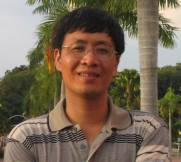Jianxian Qiu　　　　　　　　　　　　　　　　　    Professor School of Mathematical Sciences, Xiamen University 　　　　　　　 Associate editor of Journal of Computational Physics  　 School of Mathematical Sciences, Xiamen University  Xiamen, Fujian, P. R. China, 361005. jxqiu(at)xmu.edu.cn; jxqiu(at)nju.edu.cn; jxqiu(at)ustc.edu Tel:(+86-592)258-0115, Fax:(+86-592)258-0608． Current Research InterestsNumerical solutions of conservation laws and in general convection dominated problems using:Finite difference essentially non-oscillatory (ENO) methods and weighted ENO (WENO) methodsFinite element discontinuous Galerkin methods (DG)Numerical solution of Hamilton-Jacobi type equationsComputational fluid dynamicsSimulations of multi-phase flow using DG and WENO method Conferences